## Eigenvalue and Eigenvector

A matrix usually consists of many scalar elements. Can we characterize a matrix by a few numbers? In particular, our question is given a square matrix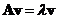, can we find a scalar number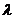and a vector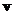such that? Any solution of equationforis called eigenvector of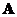. The scalar is called the eigenvalue of matrix.

Eigenvalue is also called proper value, characteristic value, latent value, or latent root. Similarly, eigenvector is also called proper vector, characteristic vector, or latent vector.

In the topic of Linear Transformation, we learned that a multiplication of a matrix with a vector will produce the transformation of the vector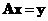. Notice the equationsaid that multiplication of a matrix by a vector is equal to multiplication of a scalar by the same vector. Thus, the scalarcharacterizes the matrix.

Since eigenvalueis the scalar multiple to eigenvector, geometrically, eigenvalue indicates how much the eigenvectoris shortened or lengthened after multiplication by the matrixwithout changing the vector orientation.

Algebraically, we can solve the equationby rearranging it into a homogeneous linear system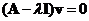where matrix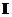is the identity matrix order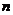. A homogeneous linear system has non trivia solution if the matrix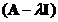is singular. That happens when the determinant is equal to zero, that is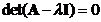. Equation is called the characteristic equation of matrix.

Expanding the determinant formula (using cofactor), we will get the solution in the polynomial form with coefficients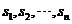. This polynomial equation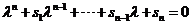is called the characteristic polynomial of matrix. The solution of the characteristic polynomial ofareeigenvalues, some eigenvalues may be identical (the same eigenvalues) and some eigenvalues may be complex numbers.

Each eigenvalue has a corresponding eigenvector. To find the eigenvector, we put back the eigenvalue into equation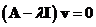. We do that for each of the eigenvalue. Ifis an eigenvector of A, then any scalar multiple is also an eigenvector with the same eigenvalue. We often use normalized eigenvector into unit vector such that the inner product with itself is one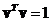.

Example:
Find eigenvalues and eigenvectors of matrix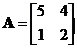Solution: we form characteristic equation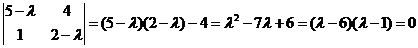The eigenvalues are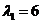and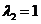.
For the first eigenvalue, the system equation is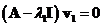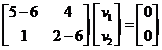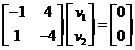The two rows are equivalent and produces equation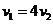. This is an equation of a line with many solutions, we can put arbitrary value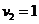to obtain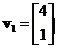. You can also write as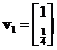or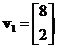and they lie on the same line.
The normalized eigenvector is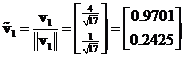For the second eigenvalue, the eigenvector is computed from the system equation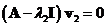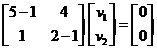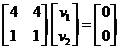The two rows are equivalent and produces equation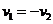. This is an equation of a line with many solutions, arbitrarily we can put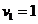to obtain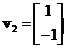. You can also write as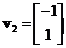or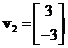and they lie on the same line.
The normalized eigenvector is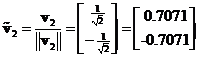Thus, eigenvaluehas corresponding eigenvector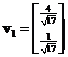and eigenvaluehas corresponding eigenvector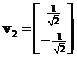.  Note that the eigenvectors are actually lines with many solutions and we put only one of the solutions. They are correct up to a scalar multiple. Since the eigenvalues are all distinct, the matrix is diagonalizable and the eigenvectors are linearly independent.

## Properties

Some important properties of eigenvalue, eigenvectors and characteristic equation are:

• Every square matrix has at least one eigenvalue a corresponding non zero eigenvector.
• When a square matrix has multiple eigenvalues (that is repeated, non-distinct eigenvalues), we have two terms to characterize the complexity of the matrix:
• The algebraic multiplicity of an eigenvalue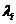is the integer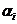associated with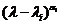when it appears in the characteristic polynomial. If the algebraic multiplicity is one, the eigenvalues is said to be simple.
• The geometric multiplicity ofis the number of linearly independent eigenvectors that can be associated with. For any eigenvalue, the geometric multiplicity is always at least one. Geometric multiplicity never exceeds algebraic multiplicity.
Example:
Matrix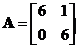has characteristic polynomial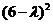thus the eigenvalue is 6 with algebraic multiplicity of 2. There is only one linearly independent eigenvector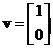, thus the geometric multiplicity is 1.
• The eigenvectors that belong to distinct eigenvalues are linearly independent eigenvectors. This is true even if the eigenvalues are not all distinct.
• If a square matrixhas fewer thanlinearly independent eigenvector, then matrixis called defective matrix. Defective matrix is not diagonalizable.
• When the eigenvalues of a square matrixare all distinct (no multiple eigenvalues), we called it non-defective matrix. A non-defective matrix haslinearly independent eigenvectors that can form a basis (i.e. a coordinate system) fordimensional space. Non-defective matrixis diagonalizable by similarity transformation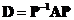into a diagonal matrix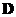. The eigenvalues oflie on the main diagonal of. Modal matrix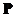is formed by horizontal concatenation of thelinearly independent eigenvectors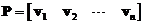.
• If matrixis symmetric then matrixhas linearly independent Eigen vectors and the Eigen values of symmetric matrixare all real numbers (no complex numbers).
• If all eigenvalues of symmetric matrixare distinct (all eigenvalues are simple), then matrixcan be transformed into a diagonal matrix. Furthermore, the eigenvectors are orthogonal.
• Matrixsatisfies its own characteristic equation. If polynomial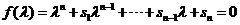is the characteristics polynomial equation of a square matrix A, then matrixsatisfies Cayley-Hamilton equation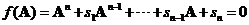.